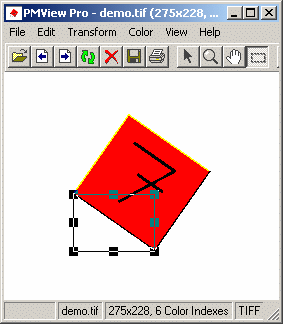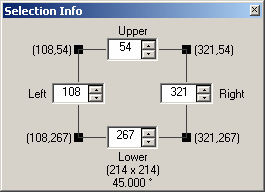# Selection Info window

Using the Selection Info window you can set and view the coordinates and size of the selected area.

The coordinates of the four corners of the selected area are indicated in the format (x,y). The origin (0,0) is at the lower-left corner. At the bottom of the window the size of the selected area is indicated as (width x height). Below the size is the transformation angle.

Use the Left, Right, Upper, and Lower controls to adjust the edges of the selection.

Example on how to use the selection rectangle to measure angles

Below, we have an image of the number seven. The number has been rotated by an unknown amount. In order to be able to turn it back again, we need to know the angle. The angle can be measured by positioning the selection rectangle (selection area) as illustrated below.Now you can read the angle directly from the Selection Info window. In this case we would need to rotate the image by 35 degrees to the right in order to turn it back. Note that the angle depends on how you measure, sometimes you may need to calculate your angle as 90 degrees minus the angle indicated by PMView.Note: If you're pasting a selection you cannot resize the area, thus the upper setting will follow the lower setting, the right will follow the left and vice versa.